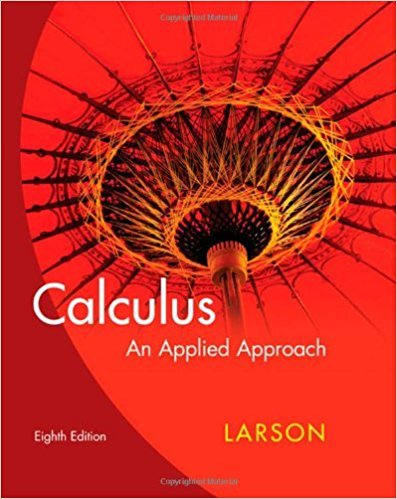×
×

# Solutions for Chapter 8.2: The Trigonometric Functions## Full solutions for Calculus: An Applied Approach | 8th Edition

ISBN: 9780618958252Solutions for Chapter 8.2: The Trigonometric Functions

Solutions for Chapter 8.2
4 5 0 244 Reviews
10
5
##### ISBN: 9780618958252

This textbook survival guide was created for the textbook: Calculus: An Applied Approach , edition: 8. Chapter 8.2: The Trigonometric Functions includes 78 full step-by-step solutions. Calculus: An Applied Approach was written by and is associated to the ISBN: 9780618958252. This expansive textbook survival guide covers the following chapters and their solutions. Since 78 problems in chapter 8.2: The Trigonometric Functions have been answered, more than 24035 students have viewed full step-by-step solutions from this chapter.

Key Calculus Terms and definitions covered in this textbook
• Dihedral angle

An angle formed by two intersecting planes,

• Ellipsoid of revolution

A surface generated by rotating an ellipse about its major axis

• Geometric sequence

A sequence {an}in which an = an-1.r for every positive integer n ? 2. The nonzero number r is called the common ratio.

• Graphical model

A visible representation of a numerical or algebraic model.

• Half-angle identity

Identity involving a trigonometric function of u/2.

• Integers

The numbers . . ., -3, -2, -1, 0,1,2,...2

• Inverse cosine function

The function y = cos-1 x

• Inverse relation (of the relation R)

A relation that consists of all ordered pairs b, a for which a, b belongs to R.

• Maximum r-value

The value of |r| at the point on the graph of a polar equation that has the maximum distance from the pole

• Multiplicative identity for matrices

See Identity matrix

• Multiplicative inverse of a real number

The reciprocal of b, or 1/b, b Z 0

• Partial fraction decomposition

See Partial fractions.

• Polynomial in x

An expression that can be written in the form an x n + an-1x n-1 + Á + a1x + a0, where n is a nonnegative integer, the coefficients are real numbers, and an ? 0. The degree of the polynomial is n, the leading coefficient is an, the leading term is anxn, and the constant term is a0. (The number 0 is the zero polynomial)

A procedure for fitting a quadratic function to a set of data.

• Reflexive property of equality

a = a

• Resolving a vector

Finding the horizontal and vertical components of a vector.

• Slant line

A line that is neither horizontal nor vertical

• Square matrix

A matrix whose number of rows equals the number of columns.

• Term of a polynomial (function)

An expression of the form anxn in a polynomial (function).

• Ymax

The y-value of the top of the viewing window.

×